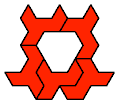# Polyomino and Polyking Tiling

## Tiling Rectangles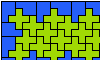Two-Pentomino Balanced Rectangles. Tile a rectangle with two pentominoes in equal quantities.Two-Pentomino Holey Balanced Rectangles. Tile a rectangle with two pentominoes in equal quantities, allowing one-cell holes.Scaled Two-Pentomino Rectangles. Tile a rectangle with two pentominoes at various sizes.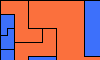Scaled Two-Pentomino Balanced Rectangles. Tile a rectangle with various sizes of two pentominoes in equal areas.Three-Pentomino Rectangles. Tile a rectangle with copies of three pentominoes.Scaled Three-Pentomino Rectangles. Tile a rectangle with three pentominoes at various sizes.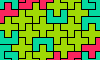Three-Pentomino Balanced Rectangles. Tile a rectangle with three pentominoes in equal quantities.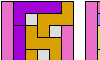Three-Pentomino Holey Balanced Rectangles. Tile a rectangle with three pentominoes in equal quantities, allowing one-cell holes.Scaled Three-Pentomino Balanced Rectangles. Tile a rectangle with various sizes of three pentominoes in equal areas.Separated Pentominoes Tiling a Rectangle. Tile the largest possible rectangle with copies of three or four pentominoes, with no two copies of the same pentomino touching.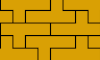Prime Rectangle Tilings for the Y Pentomino. Irreducible rectangles formed of Y pentominoes.Yin-Yang Dominoes. Arrange 10 of the 12 pentominoes to cover a bi-colored domino.Hexomino Pair Rectangles. Arrange copies of two hexominoes to form a rectangle.Scaled Hexomino Pair Rectangles. Arrange copies of two hexominoes at various scales to form a rectangle.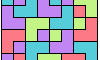Prime Rectangles for Tetrakings.. For each tetraking, find the irreducible rectangles that it can tile.

## Tiling L-Shaped Polyominoes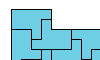Tiling an L Shape with a Polyomino. Tile an L-shaped polyomino with copies of a given polyomino.Tiling an L Shape with the 12 Pentominoes. Tile various L-shaped polyominoes with the 12 pentominoes.Tiling an L Shape with a Tetromino and a Pentomino. Tile an L-shaped polyomino with copies of a given tetromino and pentomino.L Shapes From Two Pentominoes. Form an L-shaped (hexagonal) polyomino with copies of two pentominoes, using at least one of each.L Shapes From Two Hexominoes. Form an L-shaped (hexagonal) polyomino with copies of two hexominoes, using at least one of each.Tiling an L Shape with Three Pentominoes. Tile an L-shaped polyomino with copies of three given pentominoes.

## Other Tilings and Coverings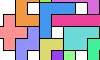Full Symmetry from the Twelve Pentominoes.Tiling a Beveled Rectangle with Polyominoes.Tiling Strips with Polyominoes. Tiling straight, bent, branched, and crossed infinite strips with polyominoes of orders 1 through 6.Uniform Polyomino Stacks. Join copies of a polyomino to make a figure with uniform row width.Perfect Polyominoes. Polyominoes that can be formed by joining all the smaller polyominoes that can tile them.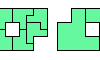Polyomino Bireptiles. Join two copies of a polyomino, then dissect the result into equal smaller copies of it.Covering a Rectangle with Copies of a Polyomino. Find the largest rectangles that copies of a polyomino can cover without overlapping.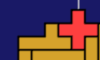A Pentomino Christmas Card. Pentomino art with an unexpected aftermath.

Back to Polyform Tiling < Polyform Curiosities
Col. George Sicherman [ HOME | MAIL ]